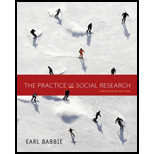Chapter 6, Problem 1RQE### The Practice of Social Research (M...

14th Edition
Earl R. Babbie
ISBN: 9781305104945

#### Solutions

Chapter
Section### The Practice of Social Research (M...

14th Edition
Earl R. Babbie
ISBN: 9781305104945
Textbook Problem

# In your own words, describe the difference between an index and a scale.

Summary Introduction

Introduction:

All scales are indices, but the reverse is not true. An index is a measurement made of other, smaller measurements, often scales. A scale is an index for only one factor.

Explanation

(This question requires a subjective answer, this is an example.) A scale measures one variable, such as temperature, while an index is comprised of scales. The wind chill index, for example, is a combination of temperature, wind speed, and measurements of how our skin deals with cold.

#### The Solution to Your Study Problems

Bartleby provides explanations to thousands of textbook problems written by our experts, many with advanced degrees!

Get Started

## Chapter 6 Solutions